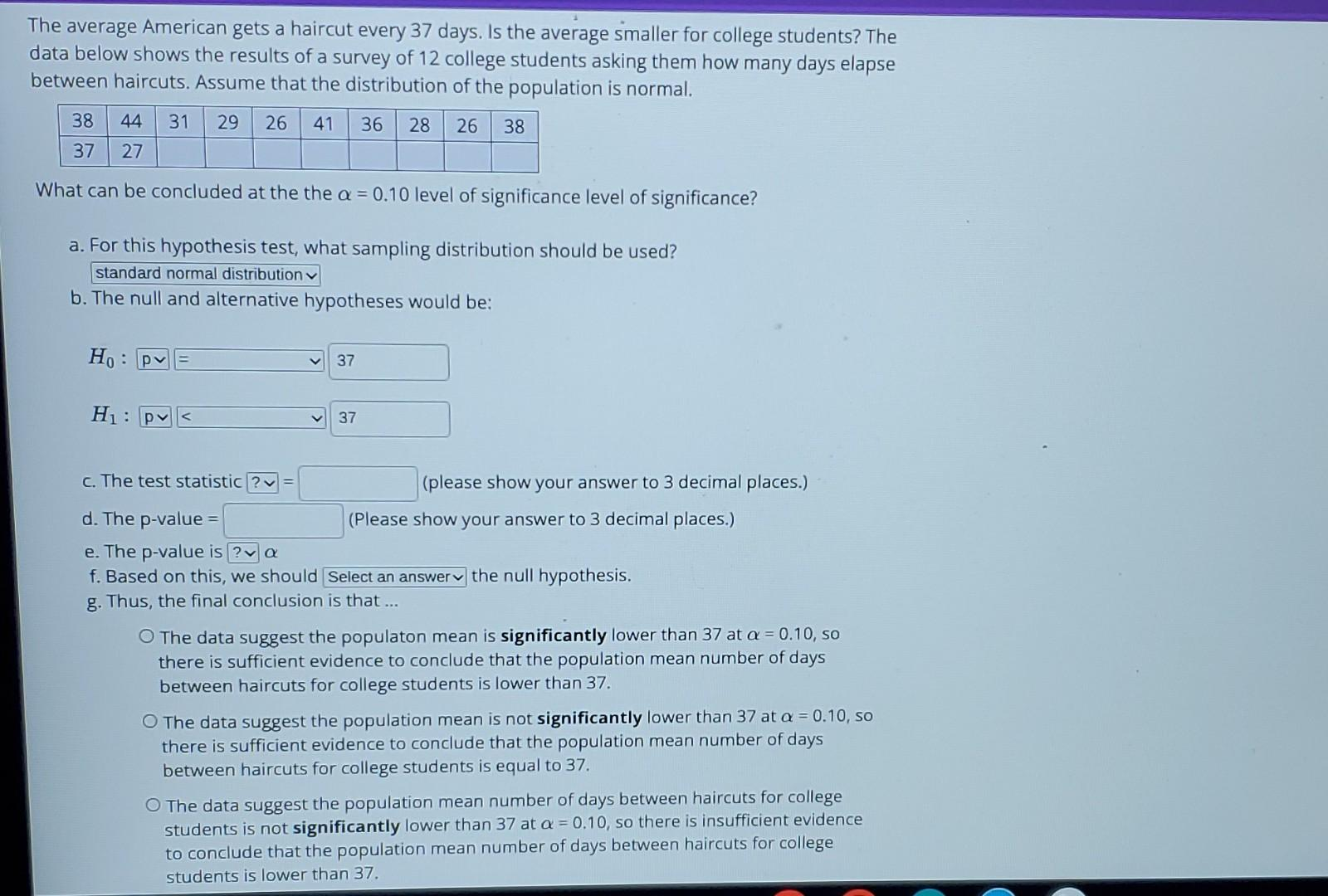# (Solved): b.,c.,d.,e.,f.,g. Please show how calculated. Thank you The average American gets a haircut ev ...b.,c.,d.,e.,f.,g.
Please show how calculated. Thank you

The average American gets a haircut every 37 days. Is the average smaller for college students? The data below shows the results of a survey of 12 college students asking them how many days elapse between haircuts. Assume that the distribution of the population is normal. What can be concluded at the the level of significance level of significance? a. For this hypothesis test, what sampling distribution should be used? standard normal distribution b. The null and alternative hypotheses would be: c. The test statistic = (please show your answer to 3 decimal places.) d. The -value (Please show your answer to 3 decimal places.) e. The p-value is f. Based on this, we should the null hypothesis. g. Thus, the final conclusion is that ... The data suggest the populaton mean is significantly lower than 37 at , so there is sufficient evidence to conclude that the population mean number of days between haircuts for college students is lower than 37 . The data suggest the population mean is not significantly lower than 37 at , so there is sufficient evidence to conclude that the population mean number of days between haircuts for college students is equal to 37. The data suggest the population mean number of days between haircuts for college students is not significantly lower than 37 at , so there is insufficient evidence

We have an Answer from Expert